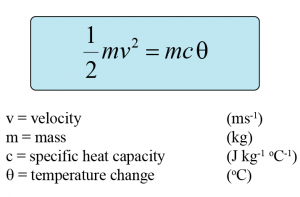# Conversion of Energy – Numerical Problems

Conversion of Gravitational Energy to Thermal Energy

mgh=mcΔθ

Example 1:
The Angel Falls in Venezuela is 979 m in height. Calculate the rise in temperature of the water when the waterfall from the top to the bottom. (Assume that all the potential energy loss of the water is converted to heat) [The specific heat capacity of water is 4200Jkg-1K-1]

The potential energy loss = mgh
The thermal energy gain of the water = mcθ

We assume that all the potential energy loss of the water is converted to heat, henceExample 2:
A mass m of lead shot is placed at the bottom of a vertical cardboard cylinder. The cylinder is 1.0 m long and closed at both ends. The cylinder is suddenly inverted so that the shot falls 1.0 m. By how much will the temperature of the shot increase if this process is repeated 200 times? Assume no heat loss to the surrounding. [The specific heat capacity of lead is 130Jkg-1K-1]

Distance fall, h = 200 x 1m = 200m
Gravitational field strength, g = 10 m/s²### Conversion of Kinetic Energy to Thermal EnergyExample 3:
A 2.5g lead bullet is moving at 200 m/s when it strikes a wooden block and is brought to rest. If all the kinetic energy  is transferred to thermal energy in the bullet, find the rise in temperature of the bullet. [The specific heat capacity of lead is 130Jkg-1K-1]

v = 200m/sConversion of Electrical Energy to Thermal Energy
Electrical energy, E = Pt

If we assume that all the electrical energy convert into thermal energy, then

Pt=mcΔθ

Example 4:
An electric heater supplies 2.5 kW of power in the form of heat to a tank of 0.02m³ of water. How long will it take to heat the water from 25 °C to 70 °C? Assume heat losses to the surroundings to be negligible. [Density of water = 1000kg/m³; The specific heat capacity of water is 4200Jkg-1K-1]